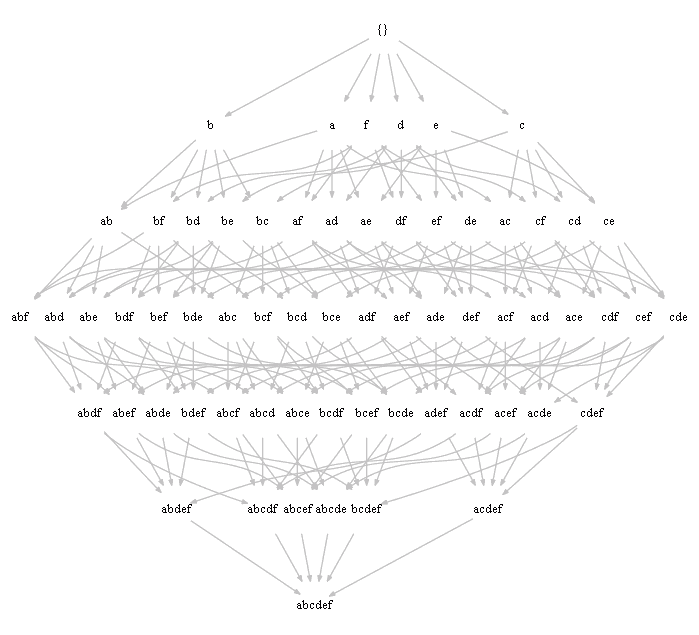# ML Wiki

## Lattice

a Lattice is a partially ordered set in which every two elements have

• a supremum (also called a least upper bound or join) and
• an infimum (also called a greatest lower bound or meet).

## Hasse Diagram

Hasse Diagram 

• a way of representing finite partially ordered sets

Layer approach

• page 33 - onwards  - software for drawing

### Drawing Powerset with Dot

Generating it in python:

from itertools import chain,combinations,product
from collections import defaultdict

# from https://docs.python.org/2/library/itertools.html#recipes
def powerset(iterable):
s = list(iterable)
return chain.from_iterable(combinations(s, r) for r in range(len(s)+1))

st = 'abcdef'
ps = {''.join(x): set(x) for x in powerset(st)}

dep = defaultdict(list)
for (s1, s2) in product(ps, ps):
if len(s1) + 1 == len(s2) and ps[s1].issubset(ps[s2]):
dep[s1].append(s2)

def join_quoted(it): return ','.join(['"%s"' % s for s in it])

for d in sorted(dep, key=len):
print ' ', '"%s"' % d, '->', join_quoted(dep[d])


Drawing it with dot:

digraph A {
node[shape=none, fontsize=10, width=0.3, fixedsize=true]
edge[arrowsize=.4,color=grey]
nodesep=0.05

"{}" -> "a","c","b","e","d","f"
"c" -> "ac","cf","ce","cd","bc"
"b" -> "ab","bd","be","bf","bc"
"e" -> "ae","ef","ce","be","de"
"f" -> "af","ef","cf","bf","df"
"ac" -> "abc","acf","ace","acd"
"ab" -> "abc","abd","abe","abf"
"ef" -> "cef","bef","aef","def"
"cf" -> "cef","acf","cdf","bcf"
"ce" -> "cde","cef","ace","bce"
"cd" -> "cde","cdf","bcd","acd"
"bd" -> "bde","abd","bdf","bcd"
"bf" -> "abf","bdf","bcf","bef"
"bc" -> "abc","bcd","bce","bcf"
"be" -> "bde","abe","bce","bef"
"cde" -> "acde","bcde","cdef"
"bef" -> "abef","bdef","bcef"
"bde" -> "bdef","abde","bcde"
"abc" -> "abcd","abce","abcf"
"abd" -> "abcd","abde","abdf"
"abe" -> "abef","abde","abce"
"abf" -> "abef","abdf","abcf"
"cef" -> "acef","cdef","bcef"
"bdf" -> "bcdf","bdef","abdf"
"cdf" -> "bcdf","acdf","cdef"
"acf" -> "acdf","acef","abcf"
"ace" -> "acde","acef","abce"
"bcd" -> "bcdf","abcd","bcde"
"bce" -> "bcde","bcef","abce"
"bcf" -> "bcdf","bcef","abcf"
"acd" -> "acde","acdf","abcd"
"abef" -> "abdef","abcef"
"bdef" -> "abdef","bcdef"
"acde" -> "abcde","acdef"
"acdf" -> "abcdf","acdef"
"acef" -> "abcef","acdef"
"abcd" -> "abcde","abcdf"
"abde" -> "abcde","abdef"
"abdf" -> "abdef","abcdf"
"bcef" -> "abcef","bcdef"
"bcde" -> "abcde","bcdef"
"bcdf" -> "bcdef","abcdf"
"cdef" -> "bcdef","acdef"
"abce" -> "abcde","abcef"
"abcf" -> "abcef","abcdf"
"abdef" -> "abcdef"
"abcef" -> "abcdef"
"bcdef" -> "abcdef"
"abcde" -> "abcdef"
"abcdf" -> "abcdef"
"acdef" -> "abcdef"
}